MATH EXPLORERS' CLUB Cornell Department of Mathematics

Ehrenfeucht-Fraïssé games

 Introduction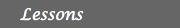1. History of EF games 2. Description 3. Examples 4. FO logic for graphs 5. EF games & FO logic 6. Applications ReferencesExamples of Ehrenfeucht-Fraïssé games. Winning Strategies.

Let us pick a very simple example in order to ilustrate the basic concepts of the EF game: Let A and B be the graphs depicted below. A is a graph on two nodes a1 and a2, and B consists of only one vertex, denoted b.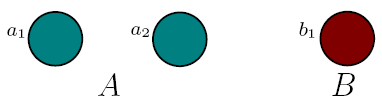Let us play 2 rounds of the EF game on A and B. The Spoiler starts the game, so he can choose any of the three nodes. If the Spoiler chooses a1 or a2, the the duplicator must pick a node in the graph B. Her only choice is the node b. If the Spoiler chooses b, then the Duplicator must select from the other graph, so she can choose either a1 or a2. This is the complete list of possible moves for the two players in the first round. Since the Duplicator makes her choice only after the Spoiler makes his, we can represent this succesion as a decision tree, depicted below. Above the red line we can read the possible moves in the first round.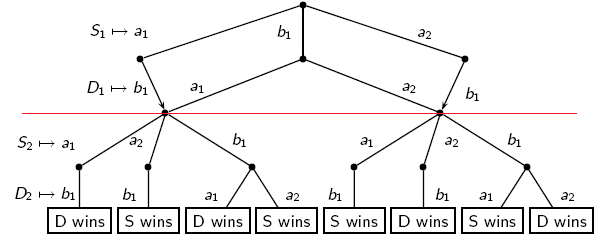The Spoiler has a couple of wining strategies for the EF game on two rounds. One strategy is the following:

• In round 1, the Spoiler chooses a1. This forces the Duplicator to choose b.
• In round 2, the Spoiler chooses a2. This forces the Duplicator to choose b.

The Spoiler wins, because the map f from {a1, a2} to {b}, f(a1)=b and f(a2)=b is not a partial isomorphism.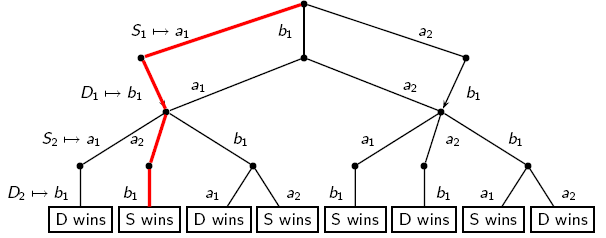Example 2:

Let us make the previous example a little bit more complicated, by considering the following graphs:If we take a look at the two graphs, we realize that they are not the same. A has a chain of 6 nodes, whereas B has a chain of 7 nodes. The question is how big are the differences between the two graphs, or, in other words, how many rounds of the EF game should we play in order for the Duplicator to loose?

One can easily see that the Duplicator has a winning strategy for the 1-round and for the 2-rounds EF game on A and B. We will show that the The Spoiler has a winning strategy for the 3-rounds EF game on A and B.

The spoiler starts by picking b4 in the first round. b4 is a special node, because it represents the middle of the chain B. According to how the Duplicator responds, the subsequent moves of the Spoiler are depicted below: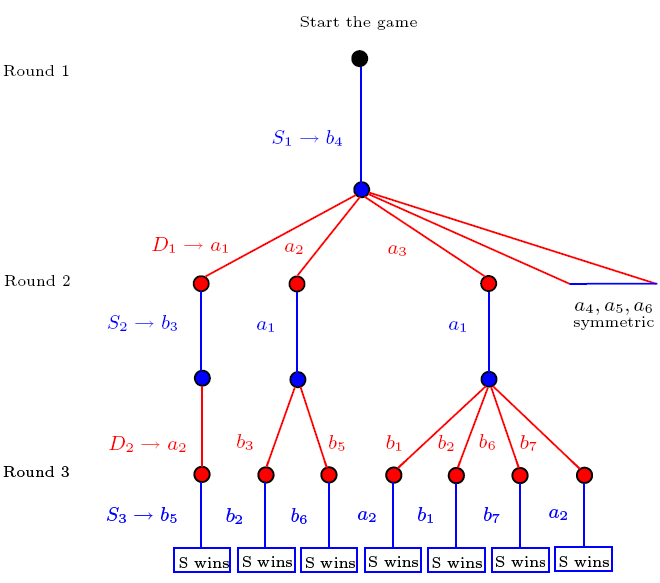Each branch of the tree represents a possible succesion of moves of the Spoiler and the Duplicator. Let us look on the branch (b4, a3, a1, b1, a2) and see why the Spoiler wins.

• Round 1:
• the Spoiler chooses b4
• the Duplicator chooses a3
• so x1 := a3 and y1 := b4
• Round 2:
• the Spoiler chooses a1
• the Duplicator chooses b1
• so x2 := a1 and y2 := b1
• Round 3:
• the Spoiler chooses a2
• the Duplicator can choose anything from B, let us denote the chosen node by b*
• so x3 := a2 and y3 := b*

After the 3 rounds, we have X = {x1, x2, x3}={a3, a1, a2} and Y = {y1, y2, y3}={b4, b1, b*}.
The map f from X to Y, f(a3) = b4, f(a1) = b1, f(a2) = b* is not a partial isomorphism. It breaks the adjacency condition, as a2 is adjacent to both a1 and a3 , whereas, whatever node the duplicator chooses in round 3 as b*, it cannot be adjacent to both b1 and b4.

The Spoiler has won by exploting the fact that in the graph B, b4 is 3 nodes away from the edges of the chain, whereas in the graph A a3 is 2 nodes away from the left edge and 3 nodes away from the right edge. The fact that the Spoiler played b4 in round 1 was very important.

Example 3:

Let A and B be the graphs from the picture. We will show that the Duplicator has a winning strategy for the 2 rounds EF game played on A and B.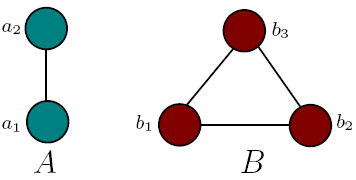Let us try to describe a winning strategy for the Duplicator:
• Round 1:
• the Spoiler can choose any node from A or B.
• if the Spoiler chooses from A, then the Duplicator will choose b1; else, the Duplicator chooses a1.
• Let us assume that the Spoiler has chosen a2. So after first round we can have x1 := a2, y1 := b1.
• Round 2:
• no matter what the Spoiler chooses, the Duplicator can mirror that. Suppose for instance that this time, the Spoiler chooses a node from the graph B.
• If Spoiler chooses b1 (same as y1 = b1), then the Duplicator must repeat its choice from round 1, and chooses a2.
• If Spoiler chooses b2 or b3(nodes adjacent to y1 = b1), then the Duplicator must choose a node adjacent to x1 = a2).
• So after the second round x2 := a2 and y2 := b1, or x2 := a1 and y2 := b2/b3.
• It is easy to check that in either case, the function f from X to Y is a partial isomorphism.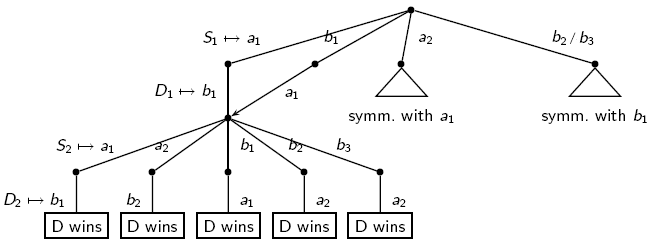Winning strategies:

A strategy for a player is a set of rules that describe exactly how that player should choose, depending on how the two players have chosen at earlier moves. A strategy for a player is said to be winning if that player wins every play in which he or she uses the strategy, regardless of what the other player does. At most one of the players has a winning strategy (since otherwise the players could play their winning strategies against each other, and both would win). There is always a winning strategy for either the Spoiler or the Duplicator. As above, we write A ~n B, if there is a winning strategy for the Duplicator for the n-rounds EF games on the graphs A and B.

A winning strategy for the Spoiler can be described as follows:
• There is a possible move for the Spoiler such that
forall possible answer moves of the Duplicator
there is a possible move for the Spoiler such that
forall possible answer moves of the Duplicator
...
after n rounds, the Spoiler wins
A winning strategy for the Duplicator can be described as follows:
• Forall possible moves of the Spoiler
there is a possible answer move of the Duplicator such that
forall possible moves of the Spoiler
there is a possible answer move of the Duplicator such that
...
after n rounds, the Duplicator wins

One can easily remark that if the Duplicator has a winning strategy for the n rounds EF game, then it also has a strategy for the k rounds EF game, where k is less then n.

Exercises

Exercise 1. Let A and B be the graphs drown below.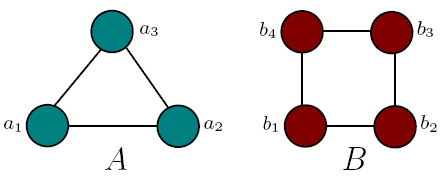1. Prove that the Duplicator has a winning strategy for the 3 rounds EF game, that is A~3B.
2. Prove that the Spoiler has a winning strategy for the 4 rounds EF game.

 Top Next lesson